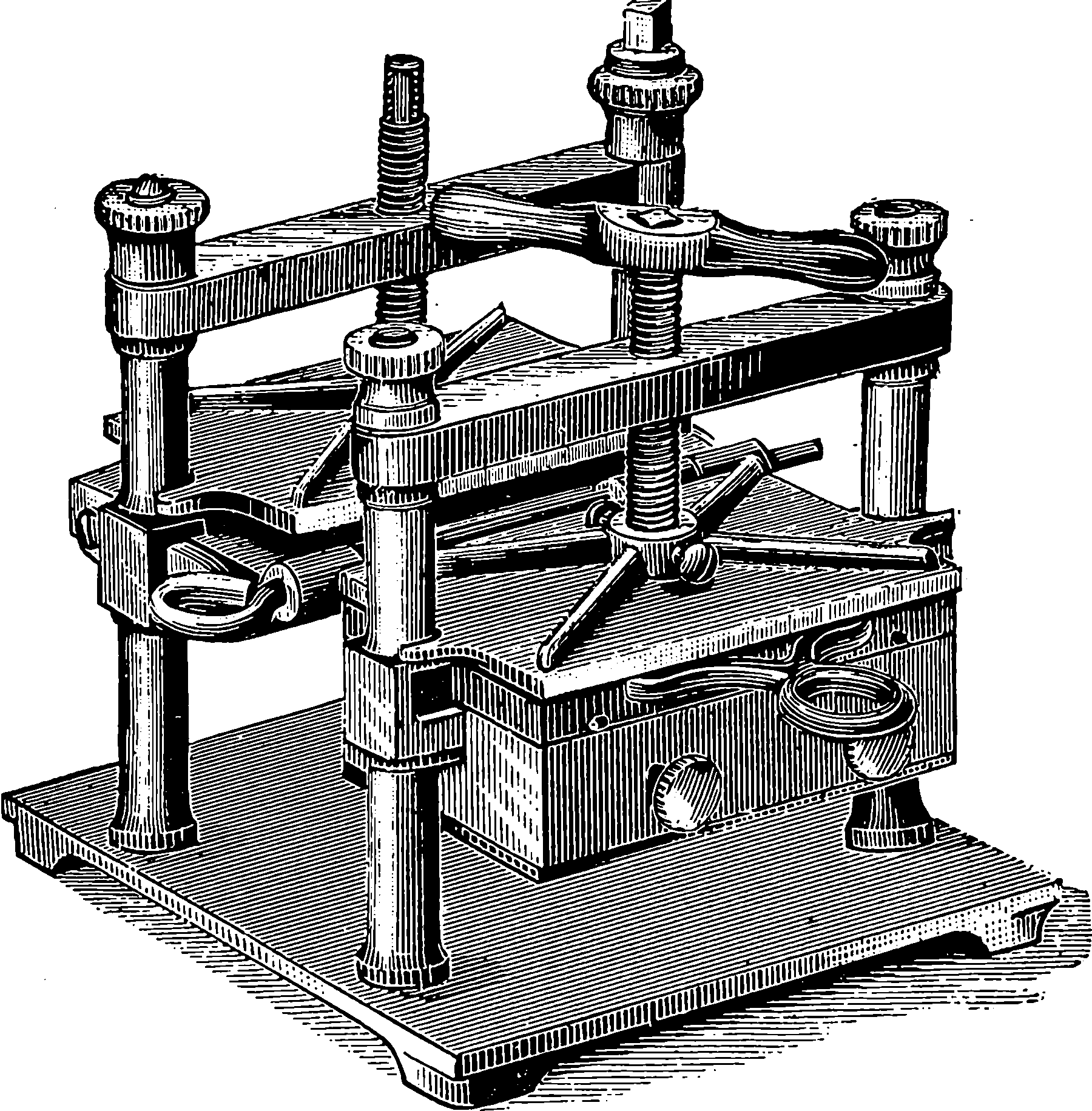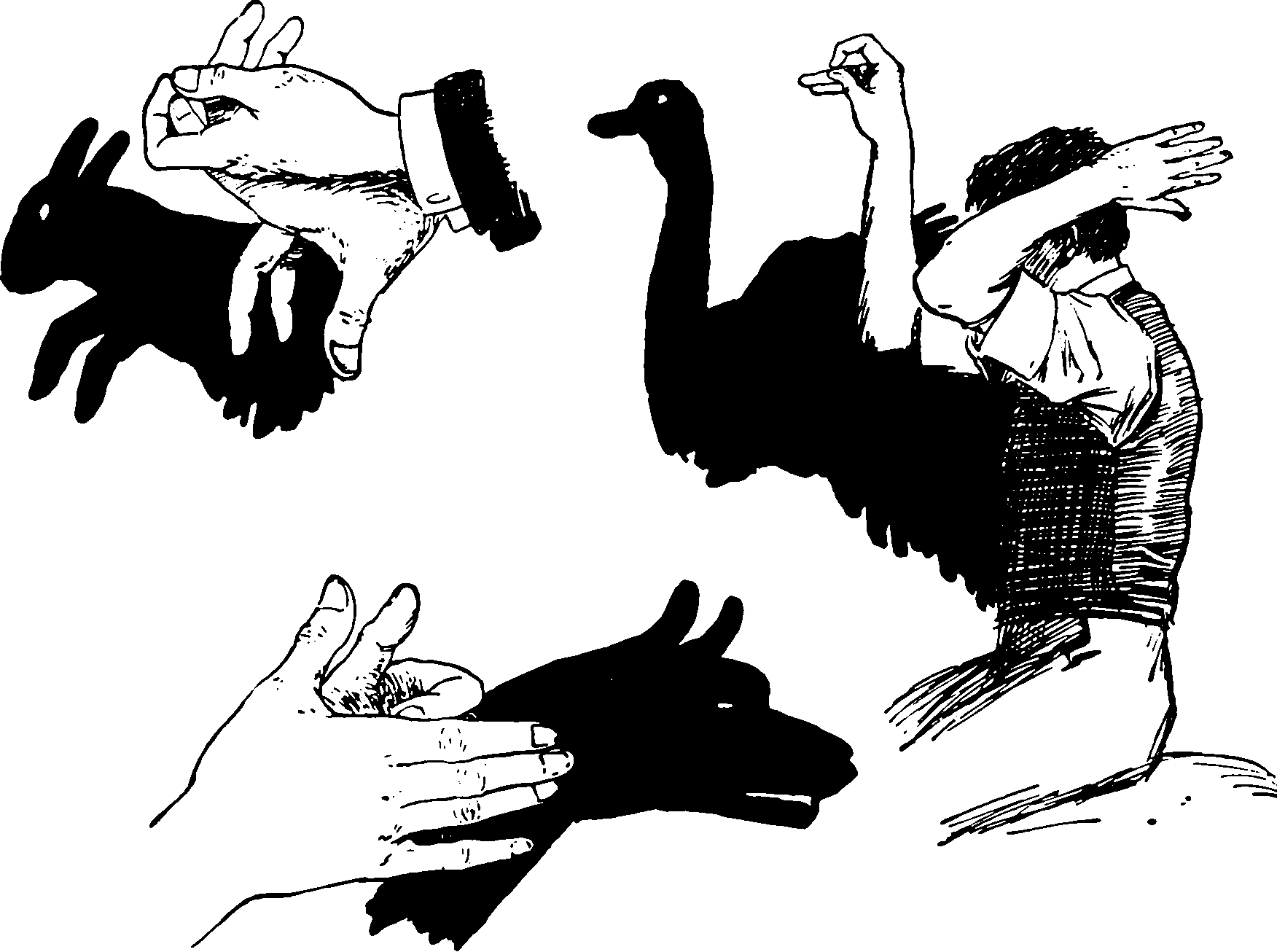# Matrix measure concentration inequalities and boundsConcentration inequalities for matrix-valued random variables.

Recommended overviews are J. A. Tropp (2015);van Handel (2017);Vershynin (2018).

## Matrix Chernoff

J. A. Tropp (2015) summarises:

In recent years, random matrices have come to play a major role in computational mathematics, but most of the classical areas of random matrix theory remain the province of experts. Over the last decade, with the advent of matrix concentration inequalities, research has advanced to the point where we can conquer many (formerly) challenging problems with a page or two of arithmetic.

Are these related?

Nikhil Srivastava’s Discrepancy, Graphs, and the Kadison-Singer Problem has an interesting example of bounds via discrepancy theory (and only indirectly probability). D. Gross (2011) is also readable, and gives results for matrices over the complex field.

## Matrix Chebychev

As discussed in, e.g. Paulin, Mackey, and Tropp (2016).

Let $$\mathbf{X} \in \mathbb{H}^{d}$$ be a random matrix. For all $$t>0$$ $\mathbb{P}\{\|\mathbf{X}\| \geq t\} \leq \inf _{p \geq 1} t^{-p} \cdot \mathbb{E}\|\mathbf{X}\|_{S_{p}}^{p}$ Furthermore, $\mathbb{E}\|\mathbf{X}\| \leq \inf _{p \geq 1}\left(\mathbb{E}\|\mathbf{X}\|_{S_{p}}^{p}\right)^{1 / p}.$

TBC.

## Matrix Efron-SteinThe “classical” Efron-Stein inequalities are simple. The Matrix ones, not so much

e.g. Paulin, Mackey, and Tropp (2016).

## Gaussian

Handy results from Vershynin (2018):

Takes $$X \sim N\left(0, I_{n}\right).$$

Show that, for any fixed vectors $$u, v \in \mathbb{R}^{n},$$ we have $\mathbb{E}\langle X, u\rangle\langle X, v\rangle=\langle u, v\rangle$

Given a vector $$u \in \mathbb{R}^{n}$$, consider the random variable $$X_{u}:=\langle X, u\rangle .$$

Further, we know that $$X_{u} \sim N\left(0,\|u\|_{2}^{2}\right) .$$ It follows that $\mathbb{E}\left[(X_{u}-X_{v})^2\right]^{1/2}=\|u-v\|_{2}$ for any fixed vectors $$u, v \in \mathbb{R}^{n} .$$

Grothendieck’s identity: For any fixed vectors $$u, v \in S^{n-1},$$ we have $\mathbb{E} \operatorname{sign}X_{u} \operatorname{sign}X_{v}=\frac{2}{\pi} \arcsin \langle u, v\rangle.$

### No comments yet. Why not leave one?

GitHub-flavored Markdown & a sane subset of HTML is supported.